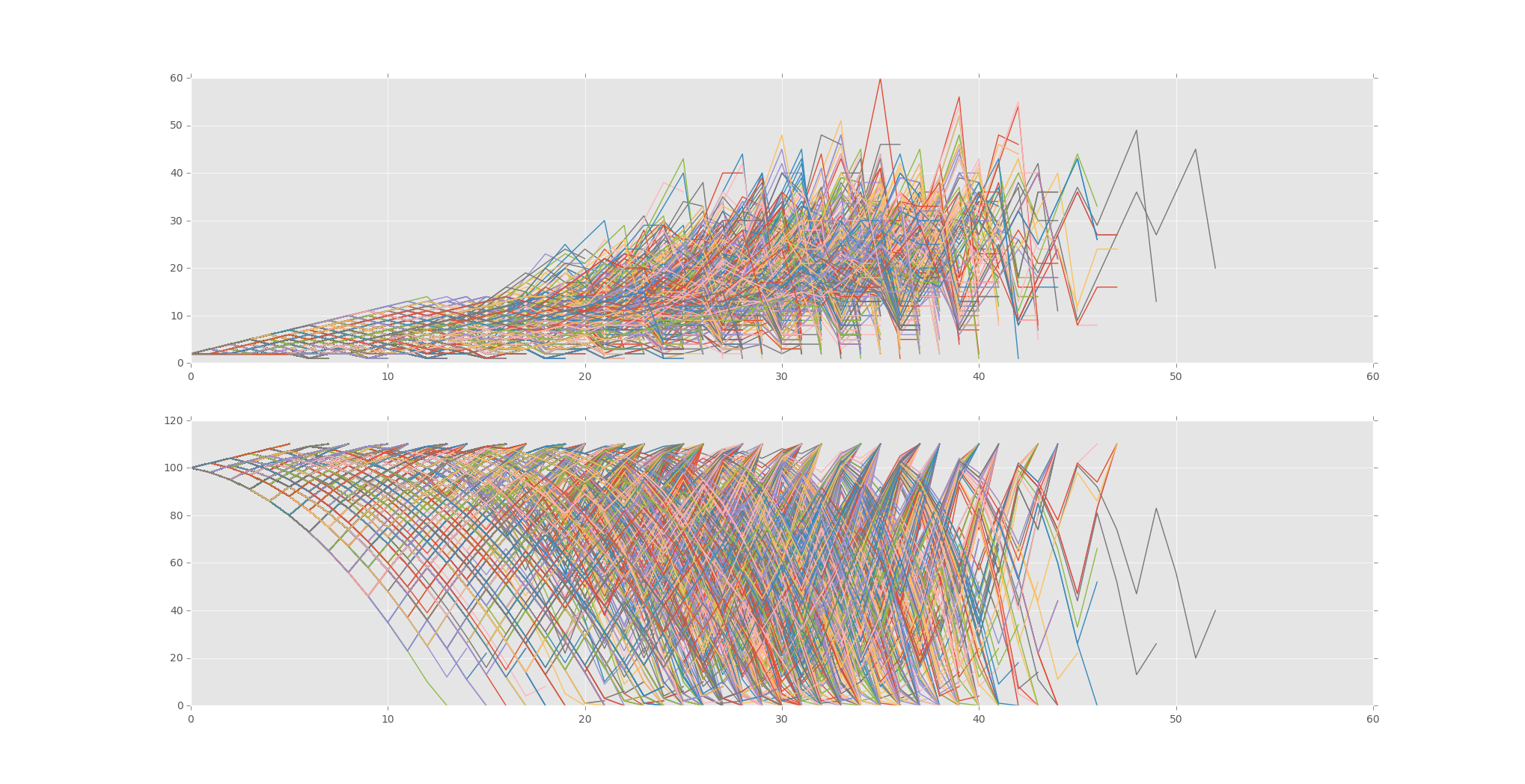## Labouchere System for Gambling Tested

```import random
import time
import matplotlib
import matplotlib.pyplot as plt
from matplotlib import style

style.use("ggplot")

broke_count = 0

totalFunded = 0
totalEnding = 0

wins = 0
losses = 0

def Labouchere():
global broke_count
global totalFunded
global totalEnding
global wins
global losses

starting_funds = 100

totalFunded += starting_funds

goal = 10
system = [1,1,1,1,1,1,1,1,1,1]
#system = [1,2,2,3,2]

profit = 0

current_funds = starting_funds

wagerSizes = []
plot_funds = []

not_broke = True

wins = 0
losses = 0

while profit < goal and not_broke:
if len(system) > 1:
size = system+system[-1]
wagerSizes.append(size)
plot_funds.append(current_funds)

else:
size = system
wagerSizes.append(size)
plot_funds.append(current_funds)

if current_funds <= 0:
not_broke = False
broke_count += 1
losses += 1

elif current_funds - size <= 0:
size = current_funds
not_broke = False
broke_count += 1

dice = random.randrange(1,101)

if dice < 51:
losses += 1
system.append(size)
current_funds -= size
profit = current_funds - starting_funds

else:
wins += 1
current_funds += size
profit = current_funds - starting_funds

if profit != goal:
try:
del system
del system[-1]
except:
pass

wagerSizes.append(size)
plot_funds.append(current_funds)

totalEnding += current_funds

s1.plot(wagerSizes)
s2.plot(plot_funds)

f = plt.figure()

sample_size = 10000

for x in range(sample_size):
Labouchere()

#print("Winners:",wins,"losers:",losses)
print(totalFunded, totalEnding)
print("Broke Percentage:", ((float(broke_count)/sample_size))*100.0)

plt.show()

```

Outcome:

```1000000 1000456
Broke Percentage: 10.41
```That's all for this tutorial series. For more Python tutorials, head to the• Monte Carlo Introduction

• Monte Carlo dice Function

• Creating a simple Bettor

• Plotting Results with Matpltolib

• Martingale Strategy

• Bettor Statistics

• More comparison

• Graphing Monte Carlo

• Fixing Debt Issues

• Analyzing Monte Carlo results

• Using Monte Carlo to find Best multiple

• Checking betting results

• D'Alembert Strategy

• 50/50 Odds

• Analysis of D'Alembert

• Comparing Profitability

• Finding best D'Alembert Multiple

• Two dimensional charting monte carlo

• Monte Carlo Simulation and Python

• Labouchere System for Gambling Tested